# Opal Image Calibration

#### Dimension Calibration

Dimension measurement variability is determined by the camera-stage geometry i.e. camera orientation and location relative to the stage. The camera needs to be oriented so that the plane of the CCD sensor is horizontal. Variation in camera to stage distance is controlled for by calibrating the size of a pixel for a given geometry. This is determined by capturing an overhead lit image of a grid with known spacing between grid lines. We have used 5 millimetre graph paper. The grid needs to be placed on the stage when it is horizontal.

In the grid image, we detect the intersection of the horizontal lines and the centre vertical line to determine the vertical line spacing, as shown in Figure 1.  Similarly the horizontal line spacing is determined from the intersection of the vertical lines and the centre horizontal line. The measurement of the horizontal and vertical line spacing in pixels allows us to determine the pixel size by comparing with the physical line spacing (5mm). GDA dimension accuracy is dependant on the accuracy of the graph paper line spacing. This is checked manually using callipers.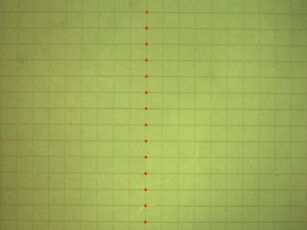Figure 1: (Left) Grid image; (Centre) Segmented horizontal lines and the centre vertical line; (Right) Vertical line spacing derived from their intersections.

#### Dimension Measurement

The length and width of the opal are determined by segmenting the backlit view of the stone as shown in Figure 2. The depth of the opal is determined by segmenting the side view of the opal obtained using the overhead lighting as shown in Figure 3. These depth measurements are averaged over several rotation angles. These dimensions are then converted from pixels to millimetres using the calibrated pixel size.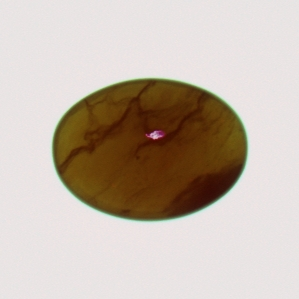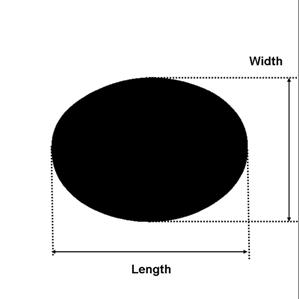Figure 2:  (Left) Backlit stone image; (Centre) Segmented stone; (Right) Length and Width measurements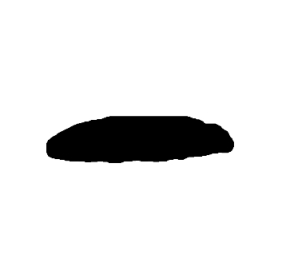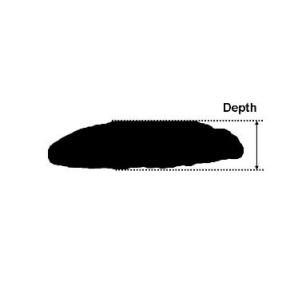Figure 3: (Left) Side view of stone using overhead lighting; (Centre) Segmented stone; (Right) Depth measurement